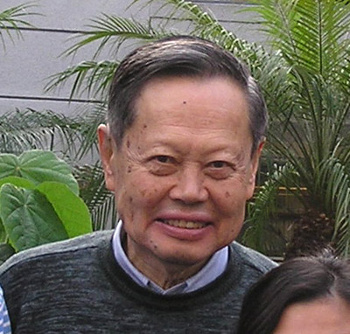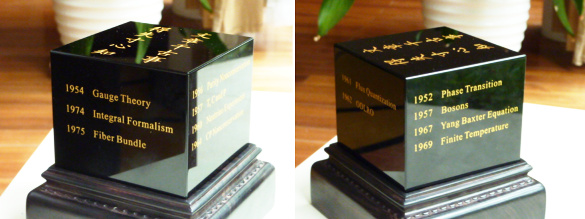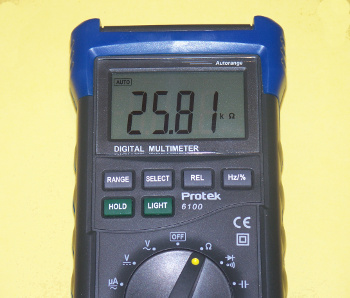### C.N. Yang

February 27, 2017

Physicist, Chen-Ning Yang, who is usually identified as "C.N. Yang" in the scientific literature, was the recipient of the 1957 Nobel Prize in Physics which he shared with fellow physicist, Tsung-dao Lee, for their prediction of parity non-conservation in processes that involve the weak nuclear force. The weak nuclear force is one of the four known fundamental forces of nature that include the strong nuclear force, gravitation, and electromagnetism.

The weak force and electromagnetism are now thought to be manifestations of a single force, the electroweak force, that will be evident at very high energies (about 100 GeV). The weak force mediates nuclear reactions that involve beta decay or alpha decay. Before Yang and Lee's theory, it was thought that such reactions would appear the same when viewed in a mirror. This parity-inversion symmetry is one of the many symmetries seen in physics. I wrote about symmetries in an earlier article (CPT Symmetry, October 29, 2015).(Via Wikimedia Commons.)

Parity-inversion symmetry is nearly always seen in beta decay, so you need to look long and hard to find the few counterexamples. A 1956 experiment involving the beta decay of cobalt-60 by physicist, Chien-Shiung Wu (1912-1997), revealed the non-conservation of parity. Non-conservation of parity means that it's possible to have an experiment distinguish left from right. One use for this would be to distinguish left from right in communication with an extraterrestrial civilization.

While it was proven that parity is not always conserved, there was hope that a combination of symmetries involving both charge (C) and parity (P) might still hold, but James Cronin and Val Fitch found CP violation in neutral kaon decay in 1964. The reason why our universe is made of matter, and not an equal mixture of matter and antimatter, might be explained by CP violation.

Presently, it appears that adding time-reversal (T) symmetry into the mix for a combined CPT symmetry is a valid symmetry. The consequence of CPT symmetry is that an antimatter universe (C) that's a mirror image of our own (P) in which particle momentum is reversed (T) would have the same laws of physics that we observe. CPT has been confirmed in all experiments to date; but, as can be imagined, such experiments have become much more difficult than Wu's 1956 experiment that demolished P symmetry.

Yang, who was born on born on October 1, 1922, was a young man in his mid-30s when he published his theory of the weak interaction, and he did much interesting work after that. On the occasion of his 90th birthday in 2012, he was presented with a black "cube" of dimensions 8 cm x 8 cm x 6.6 cm engraved with a birthday congratulation, two lines in Chinese from Tu Fu (712-770), "A piece of Literature is meant for the millennium, but its ups and downs are known already in the author's heart," and a list of his thirteen significant contributions to statistical mechanics, condensed matter physics, particle physics, and field theory, ordered by date (see figure).The presentation to C.N. Yang on the occasion of his 90th birthday. (Modified arXiv image from Ref. 1.)[1)]

Just posted on arXiv is a copy of a paper published in honor of that occasion by Yu Shi in a 2014 issue of the International Journal of Modern Physics A. Here's the list of Yang's 13 significant contributions, as engraved on the cube, listed by topic area and year.

Statistical Mechanics
1952-Phase Transition
1957-Bosons
1967-Yang-Baxter Equation
1969-Finite Temperature
Condensed Matter Physics
1961-Flux Quantization
1962-Off-Diagonal Long-Range Order
Particle Physics
1956-Parity Nonconservation
1957-T, C and P
1960-Neutrino Experiment
1964-CP Nonconservation
Field Theory
1954-Gauge Theory
1974-Integral Formalism
1975-Fiber Bundle

While I'm not skilled in particle physics and not comfortable reading papers that are mathematically dense, I did work in the field of superconductivity for a time. In this area of condensed matter physics, Yang and co-author, Nina Byers, explained the magnetic flux quantization in superconducting rings observed at Stanford University (Stanford, CA) by Bascom S. Deaver and William M. Fairbank.[3-4] In such rings, the magnetic flux is quantized in units of hc/2e, where h is the Planck constant, c is the speed of light, and e is the electric charge. The quantum of magnetic flux is 2.0678 x 10-15 weber.

One important feature of this equation is the factor of two. The BCS theory of superconductivity, published in 1957, predicted that supercurrent is carried by electron pairs, called Cooper pairs. While this theory explained many unusual features of superconductivity, such as the isotope effect, flux quantization very dramatically provided evidence for Cooper pairs.

The quantum of magnetic flux can be measured to great precision using Josephson junction devices. Combining such a measurement with the quantum Hall effect allows a very accurate estimate of the Planck constant. The quantum Hall effect gives a value for an electrical resistance RK called the von Klitzing constant, RK=h/(e2). It's interesting that there's a quantum of electrical resistance, ≈25812.80756 ohms, but it's more important that a combination of precise measurements will yield an excellent calculated value for h.Not NIST precision

The quantum resistance value, about 25.81 kohm, as simulated with a variable resistor and measured on a digital multimeter.

(Photo by author).)### References:

Linked Keywords: Physicist; Chen-Ning Yang; scientific literature; Nobel Prize in Physics; Tsung-dao Lee; parity; conservation law; non-conservation; weak interaction; weak nuclear force; fundamental interaction; fundamental forces of nature; strong interaction; strong nuclear force; gravity; gravitation; electromagnetism; electroweak interaction; electroweak force; energy; energies; electronvolt; GeV; nuclear reaction; beta decay; alpha decay; theory; mirror; symmetry; physics; decade; Wikimedia Commons; counterexample; Wu experiment; cobalt-60; Chien-Shiung Wu (1912-1997); experiment; relative direction; left; right; communication; extraterrestrial civilization; charge; James Cronin; Val Fitch; CP violation; neutral kaon; radioactive decay; baryogenesis; matter; antimatter; T-symmetry; time-reversal symmetry; CPT symmetry; momentum; physical law; laws of physics; youth; young man; birthday; cube; centimeter; cm; engraved; Chinese language; Du Fu; Tu Fu (712-770); literature; millennium; author; statistical mechanics; condensed matter physics; particle physics; quantum field theory; arXiv; International Journal of Modern Physics A; phase transition; boson; Yang-Baxter equation; thermal quantum field theory; finite temperature; magnetic flux quantum; flux quantization; coherence; off-diagonal long-range order; Cowan-Reines neutrino experiment; gauge Theory; Mills Lagrangian; integral formalism; fiber bundle; mathematics; superconductivity; Nina Byers; magnetic flux quantization; torus; ring; Stanford University (Stanford, CA); Bascom S. Deaver; William M. Fairbank; magnetic flux; quantization; quantize; Planck constant; speed of light; electric charge; quantum mechanics; quantum; weber; equation; BCS theory of superconductivity; supercurrent; electron; Cooper pair; isotope effect; Josephson effect; Josephson junction device; quantum Hall effect; electrical resistance; Klaus von Klitzing; von Klitzing constant; National Institute of Standards and Technology; NIST; precision; ohm; kohm; potentiometer; variable resistor; digital multimeter.